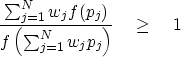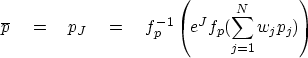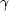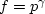Next: Additivity of envelope entropy Up: RELATED CONCEPTS Previous: Prior and posterior distributions

## Jensen average

Physicists speak of maximizing entropy, which, if we change the polarity, is like minimizing the various Jensen inequalities. As we minimize a Jensen inequality, the small values tend to get larger while the large values tend to get smaller. For each population of values there is an average value, i.e., a value that tends to get neither larger nor smaller. The average depends not only on the population, but also on the definition of entropy.

Commonly, the pj are positive andis an energy. Typically the total energy, which will be fixed, can be included as a constraint, or we can find some other function to minimize. For example, divide both terms in (3) by the second term and get an expression which is scale invariant; i.e., scaling p leaves (15) unchanged:(15)
Because the expression exceeds unity, we are tempted to take a logarithm and make a new function for minimization:(16)

Given a population pj of positive variants, and an inequality like (16), I am now prepared to define the Jensen average''.Suppose there is one element, say pJ, of the population pj that can be given a first-order perturbation, and only a second-order perturbation in J will result. Such an element is in equilibrium and is the Jensen average:(17)
Let fp denote the derivative of f with respect to its argument. Inserting (16) into (17) gives(18)
Solving,(19)

But where do we get the function f, and what do we say about the equilibrium value? Maybe we can somehow derive f from the population. If we cannot work out a general theory, perhaps we can at least find the constant,assuming the functional form to be.Next: Additivity of envelope entropy Up: RELATED CONCEPTS Previous: Prior and posterior distributions
Stanford Exploration Project
10/21/1998